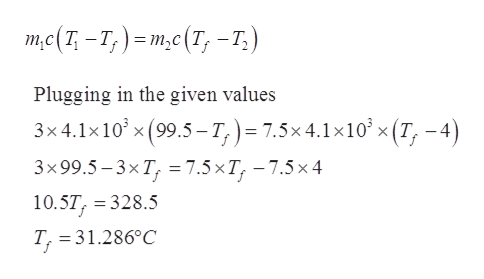# A 3 Kg mass of water is heated to a temperature of 99.5 C at 1 bar of pressure.   It is slowly poured into a heavily insulated beaker containing 7.5 Kg of water at a temperature and pressure of 4 C, 1 bar, respectively.  The specific heat of the water, an incompressible material is, c = 4.1 KJ/(Kg K).  The beakers are an adiabatic system.  The two water mass’ reach an equilibrium temperature.  There is no kinetic or potential energy change in this problem.Using the first law determine the final temperature of the water in the beaker.Calculate the entropy production in this process. (Hint: note that this is an incompressible material which means that the density and specific volume are constant)

Question

A 3 Kg mass of water is heated to a temperature of 99.5 C at 1 bar of pressure.   It is slowly poured into a heavily insulated beaker containing 7.5 Kg of water at a temperature and pressure of 4 C, 1 bar, respectively.  The specific heat of the water, an incompressible material is, c = 4.1 KJ/(Kg K).  The beakers are an adiabatic system.  The two water mass’ reach an equilibrium temperature.  There is no kinetic or potential energy change in this problem.

1. Using the first law determine the final temperature of the water in the beaker.
2. Calculate the entropy production in this process. (Hint: note that this is an incompressible material which means that the density and specific volume are constant)

check_circleExpert Solution
Step 1

The mass of water m1 = 3kg

The water is heated to a temperature of T1 = 99.5oC

The mass of water in the insulated beaker m2 = 7.5 kg

The temperature of the water inside the beaker T2 = 4oC

The pressure inside the insulated beaker P2 = 1 bar

The specific heat of water cp = 4.1 KJ/kgK

Step 2

The final temperature of water can be calculated with the help of First law of thermodynamics. The first law of thermodynamics is the law of conversation of energy for the thermodynamic systems. According to the conservation of energy, the energy given by one system is received by another system if there is no loss to the surrounding.

The energy balance equation giveshelp_outlineImage Transcriptioncloseте(1-т,) -т,c(T, -т,) Plugging in the given values 3x4.1x10 x(99.5-T,) 7.5x4.1x10x (T, -4) 3x99.5-3xT, %3D7.5 хT, —7.5х 4 10.5T, 328.5 T, 31.286°C fullscreen
Step 3

Part (b)

The entropy production in the process:

First calculate the entrop...

### Want to see the full answer?

See Solution

#### Want to see this answer and more?

Solutions are written by subject experts who are available 24/7. Questions are typically answered within 1 hour*

See Solution
*Response times may vary by subject and question
Tagged in

### Mechanical Engineering